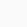# How to use intersection(∩) symbol in LaTeX?

SymbolIntersection
Type of symbolMathematics
Package (requirement)No
ArgumentNo
Latex command\cap
ExampleSi \cap Sk → Si ∩ Sk

The intersection defines the common section between the two sets. In the case of LaTeX, \cap and \bigcap commands are used to represent this symbol.

There are also other packages that allow you to print intersection symbols in documents.

\documentclass{article}
\begin{document}
$S_i \cap S_k$
$S_1 \cap S_2 = \emptyset$
$\bigcap_{i=1}^k F_i$
$\bigcap\limits_{i=1}^n S_i$
\end{document}

Output :Scroll to Top